Function Repository Resource:

# ArithmeticD

Compute the arithmetic derivative of a rational number

Contributed by: Shenghui Yang (Wolfram Research)
 ResourceFunction["ArithmeticD"][r] find the arithmetic derivative r' of a rational number r.

## Details

Arithmetic derivative uses the explict formula of integer factorization from Barbeau's "Remarks on an arithmetic derivative".
The algorithm for finding the arithmetic derivative has the same complexity as integer factoriztion.
The arithmetic derivatives of 0 and 1 are zero.
The arithmetic derivative of any prime number is 1.
Arithmetic derivative follows Leibniz rule.
The second arithmetic derivative is the arithmetic derivative of the first arithmetic derivative; higher-order arithmetic derivatives are defined analogously.
Unlike the usual derivative studied in college calculus class, the arithmetic derivative does not obey linearity in general.
The arithmetic derivative behaves in ways that are not well understood in number theory; higher order derivatives present many unresolved properties.

## Examples

### Basic Examples (2)

Find the arithmetic derivative of 100:

 In:=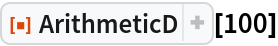Out=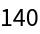Find the arithmetic derivative of the fraction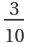:

 In:=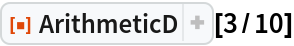Out=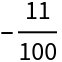### Scope (2)

Arithmetic derivate for any prime is 1:

 In:=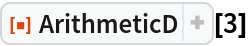Out=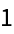Using Leibniz rule it is easy to find the formula for prime tower :

 In:=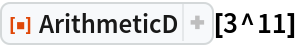Out=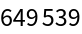which is the same as :

 In:=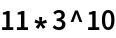Out=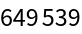### Properties and Relations (4)

Higher order arithmetic derivatives are defined successively by nesting the same operation multiple times:

 In:=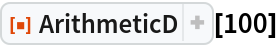Out=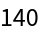In:=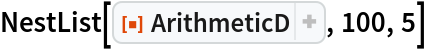Out=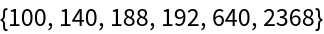Arithmetic derivative of an integer n has the following bounds: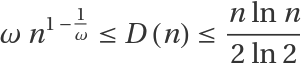, where is PrimeOmega[n] and gives the number of prime factors counting multiplicities.

The equality of the lower bound holds if and only if :

 In:=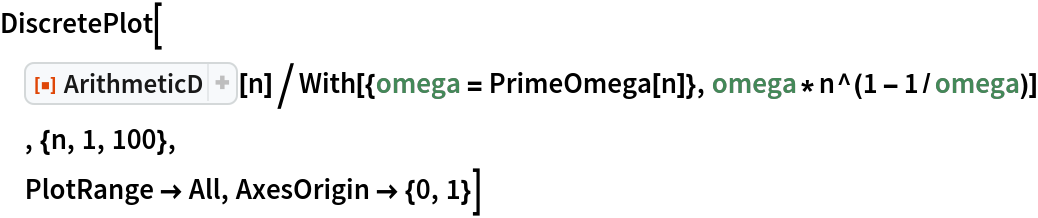Out=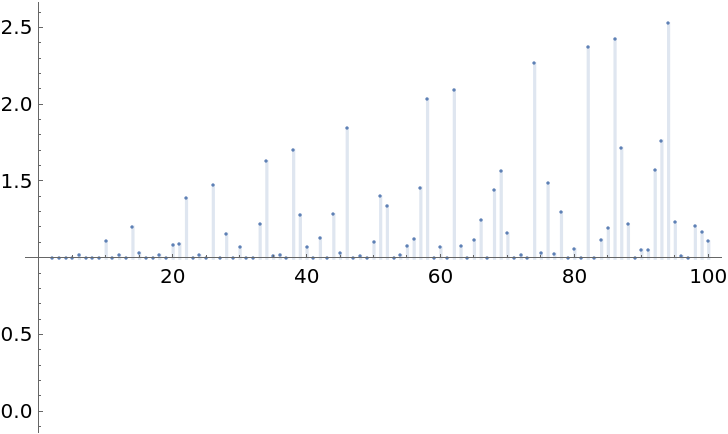The equality of the upper bound holds if and only if n is prime or a prime tower:

 In:=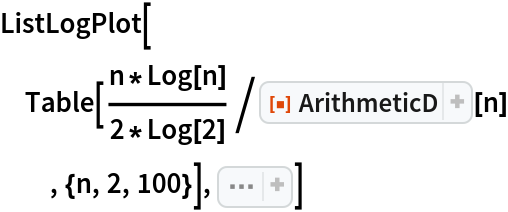Out=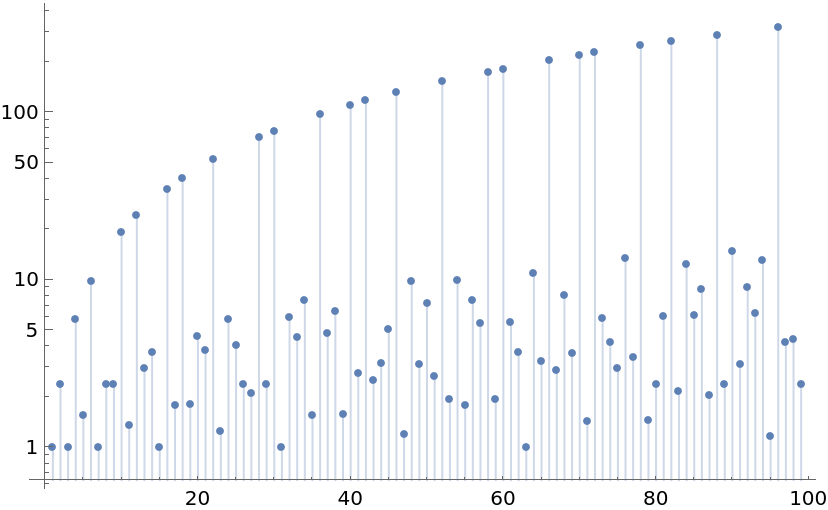if and only if where p is any prime:

 In:=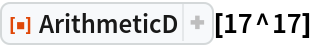Out=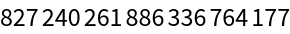In:=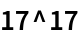Out=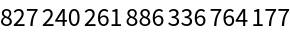It is easy to see that is not a fixed point:

 In:=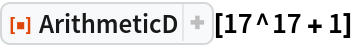Out=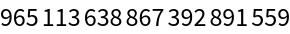has nontrivial solution for some rational value x:

 In:=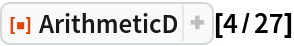Out=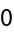### Possible Issues (2)

The arithmetic derivative does not obey linearity in general:

 In:=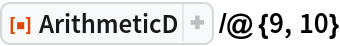Out=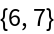Clearly 1 is not same as :

 In:=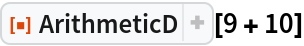Out=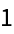However, if we find some m and n such that , then for all integer k. For instance:

 In:=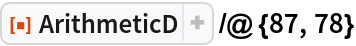Out=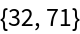In:=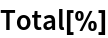Out=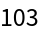In:=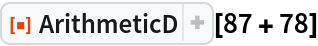Out=Then the following also true:

 In:=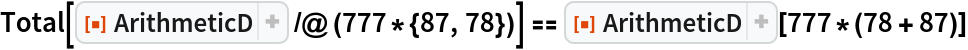Out=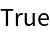### Neat Examples (4)

The graph of for

 In:=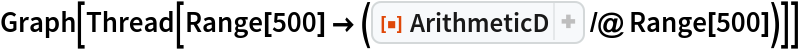Out=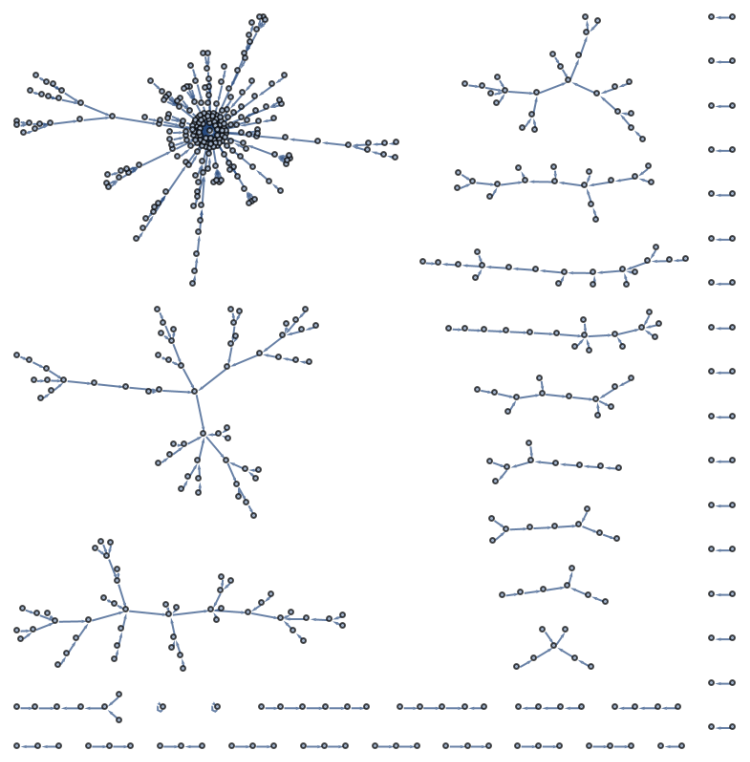We can define the arithmetic integral by solving the equation given a, . The algorithm can be implemented with simple search in the interval found in the aforementioned bounds: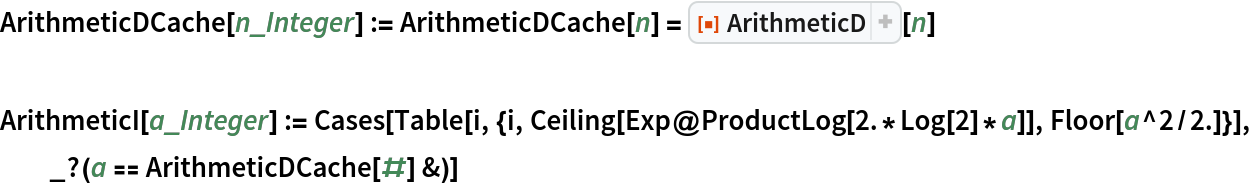For example, the integrals of 10 and 100:

 In:=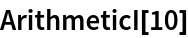Out=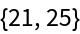In:=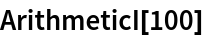Out=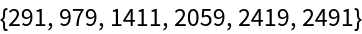We also can find the a's that do not have their integrals less than 1000: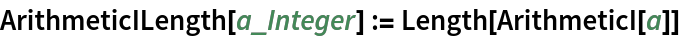In:=Out=Here is a list of number with more than one integral:

 In:=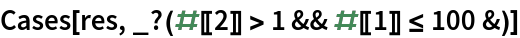Out=The result means that 10 has two integrals, 12 has two integrals and so on.

The Goldbach conjecture implies that the differential equation has a positive integer solution for any natural number :

 In:=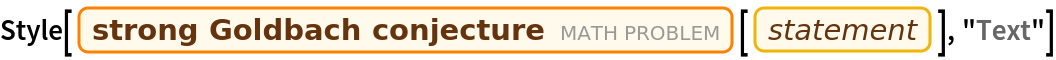Out=For instance as a sum of two primes:

 In:=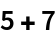Out=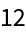By the Leibniz rule of arithmetic derivative, 35 is one solution for n such that the differential equation holds: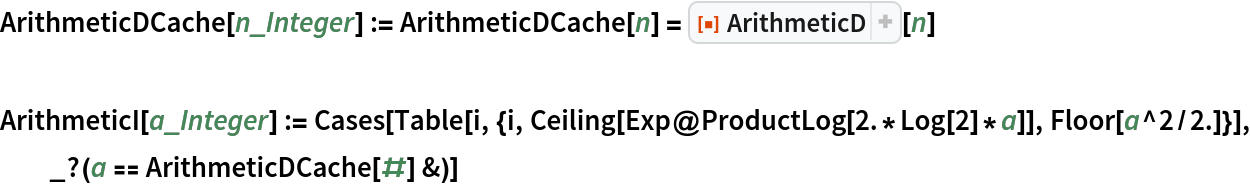In:=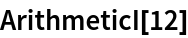Out=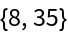Twin prime conjecture implies that the differential equation has infinite number of solutions:

 In:=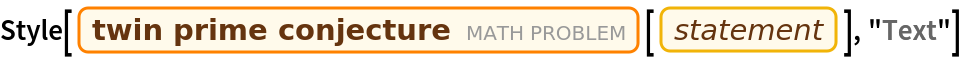Out=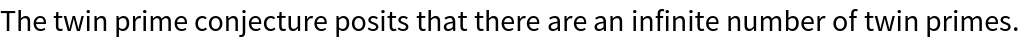This is from the application of Leibniz rule on the arithmetic derivative of where p is any prime:

 In:=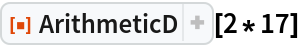Out=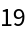In:=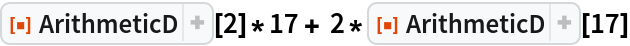Out=In general, . If is a prime again,

 In:=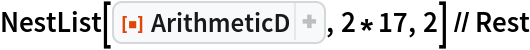Out=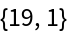Thus we shall have infinite such if the conjecture holds.

Shenghui Yang

## Version History

• 1.0.1 – 15 June 2022
• 1.0.0 – 07 June 2022